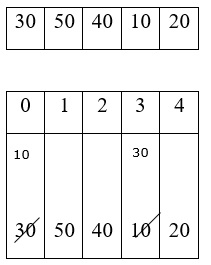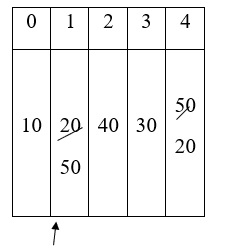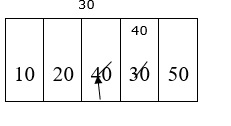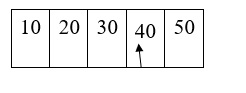# Explain the procedure of selection sort in C language

The selection Sort is an assaulting algorithm that works to find the smallest number from an array and then place it to the first position. The next array that is to be traversed will start from index, next to the position, where the smallest number is placed.

## Procedure of selection sort

• Select the first smallest element among the list of elements and place it in the first position.

• Repeat the same for remaining elements in the list until all the elements gets sorted.

Consider the following list −### First Pass

Sm = a = 30 Sm

a < sm $\square$ $\square$ 50 <30 (F) $\square$ $\square$ 30

a < sm $\square$ $\square$ 40 <30 (F) $\square$ $\square$ 20$\square$ exchange a with sm value

a < sm $\square$ $\square$ 10 <30 (T) $\square$ $\square$ 10

a < sm $\square$ $\square$ 20<10 (F) $\square$ $\square$ 10

10 50 40 30 20

### Second passSm = a = 50 sm

a < sm $\square$ $\square$ 40 <50 (T) $\square$ 40 $\square$

a < sm $\square$ $\square$ 30 <40 (T) $\square$ 30 exchange a with sm value

a < sm $\square$ $\square$ 20<30 (T) $\square$ 20

10 20 40 30 50

### Third PassSm = a = 40 Sm

a < sm $\square$ $\square$ 30 <40 (T) $\square$ $\square$ 40

a < sm $\square$ $\square$ 50<40 (F) $\square$ $\square$ 30 exchange a with sm value

10 20 30 40 50

### Fourth passSm = a = 40 Sm

a < $\square$ $\square$ sm 50 <40 (F) $\square$ 40 $\square$ exchange a with sm value

### Procedure

Refer the procedure given below for selection sorting.

for (i=0; i<n-1; i++){
sm=i;
for (j=i+1; j<n; j++){
if (a[j] < a[sm])
sm=j;
}
t=a[i];
a[i] = a[sm];
a[sm] = t;
}
}

## Example

Following is the C program for selection sorting technique −

#include<stdio.h>
int main(){
int a, i,j,n,t,sm;
printf("enter the No: of elements in the list:");
scanf("%d", &n);
printf("enter the elements:");
for(i=0; i<n; i++){
scanf ("%d", &a[i]);
}
for (i=0; i<n-1; i++){
sm=i;
for (j=i+1; j<n; j++){
if (a[j] < a[sm]){
sm=j;
}
}
t=a[i];
a[i]=a[sm];
a[sm]=t;
}
printf ("after selection sorting the elements are:");
for (i=0; i<n; i++)
printf("%d\t", a[i]);
return 0;
}

## Output

When the above program is executed, it produces the following result −

enter the No: of elements in the list:
4
enter the elements:
45
12
37
68
after selection sorting the elements are:
12 37 45 68